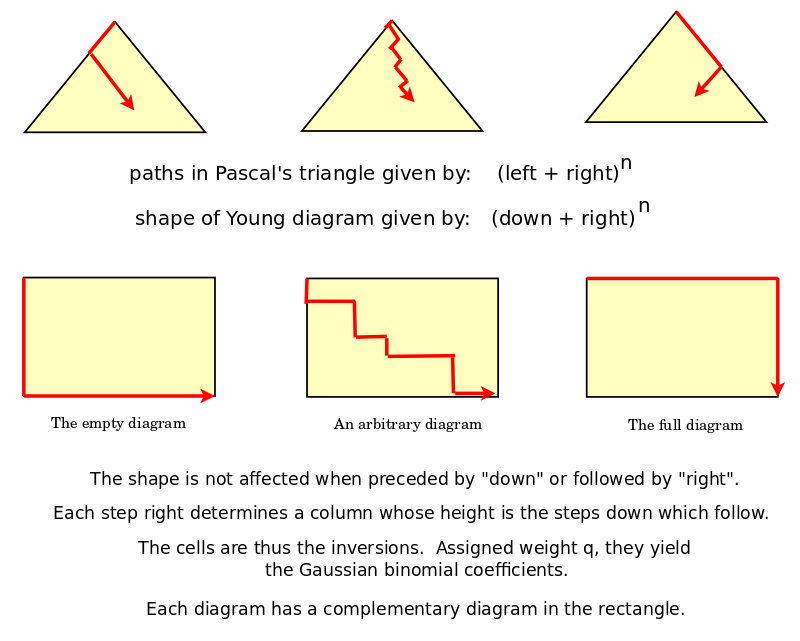# Book: YoungDiagramsAsPaths

Solved: Identify Young diagrams as paths in Pascal's triangle.

Each location (n i) in Pascal's triangle can be identified with the Young diagrams that fit in a rectangle with sides of length i and n-i.

Thus a Young diagram can be identified with a smallest location (n i) and all locations (m j) for which j>=i and m-j >= n-i, which is to say, for all in the triangle below (n i).Discussion

Note that each cell in the rectangle gives the choice, across terms, of x-choice-left vs. y-choice-right. So we can thus infer choices - how we would have chosen - given the choices available, and given the choices made. Inversions are related to a change in choice, in preference, and thus they provide information about changes in the chooser. Extrinsic vs. intrinsic symmetry issues become relevant.

Given an initial infinite sequence: ...down-down-down-right-right-right... consider the possible (finite) ways that it could be perturbed, for example: ...down-down-down-right-right-down-right-right-right... Such perturbations are the paths, or equivalently, the Young diagrams.

Parsiųstas iš http://www.ms.lt/sodas/Book/YoungDiagramsAsPaths
Puslapis paskutinį kartą pakeistas 2019 rugpjūčio 22 d., 18:04